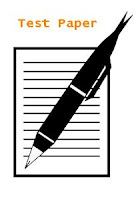## Sets

(Unit Test Paper)
CBSE Class 11 - Mathematics

1. Write the set of all vowels in the English alphabet which precede k. 

2. Is pair of sets equal? Give reasons. 
A = {2, 3} B = x : x is solution of x 2 + 5x + 6 = 0}

3. Write the following intervals in set builder form: 
(-3, 0) and [6, 12]

4. If X = {a, b, c, d} 
Y = {f, b, d, g}
Find X – Y and Y - X

5. If A and B are two given sets, Then represent the set (A – B )′ using Venn diagram. 

6. A and B are two sets such that n (A – B) = 20 + x, n (B – A) = 3x and n (A ∩ B) = x + 1. Draw a Venn diagram to illustrate this information. If n (A) = n (B), Find 
(i) the value of x
(ii) n (A ∪ B)

7. If A and B are two sets such that A ∪ B= A ∩ B, then prove that A = B 

8. Prove that if A ∪ B = C and A ∩ B = φ then A = C – B 

9. In a survey of 100 students, the no. of students studying the various languages were found to be English only 18, English but not Hindi 23, English and Sanskrit 8, English 26, Sanskrit 48, Sanskrit and Hindi 8, no language 24. Find
(i) How many students were studying Hindi?
(ii) How many students were studying English and Hindi? 

10. In a class of 50 students, 30 students like Hindi, 25 like science and 16 like both. Find the no. of students who like
(i) Either Hindi or science

(ii) Neither Hindi nor science.#### 1 comment:

1.